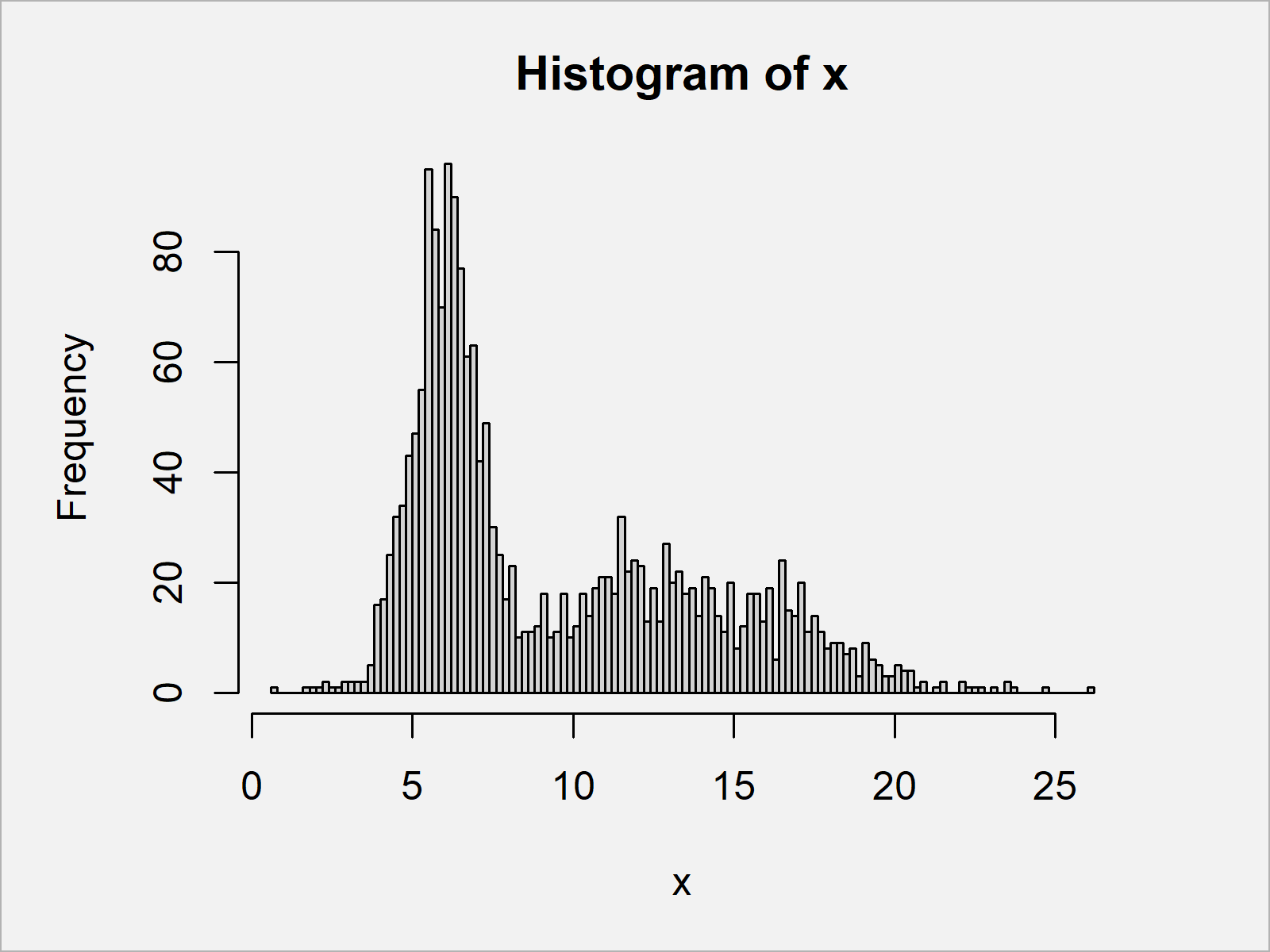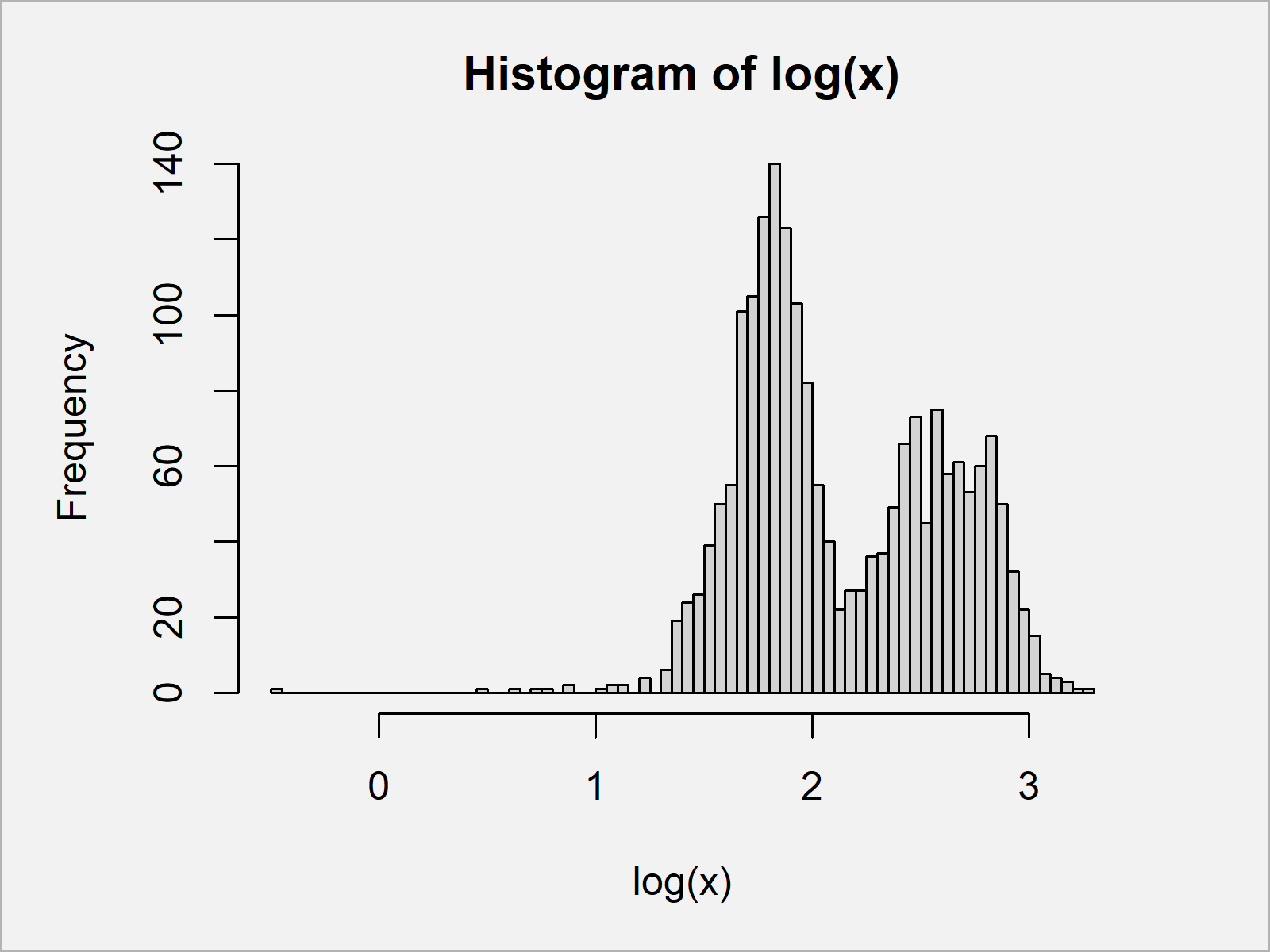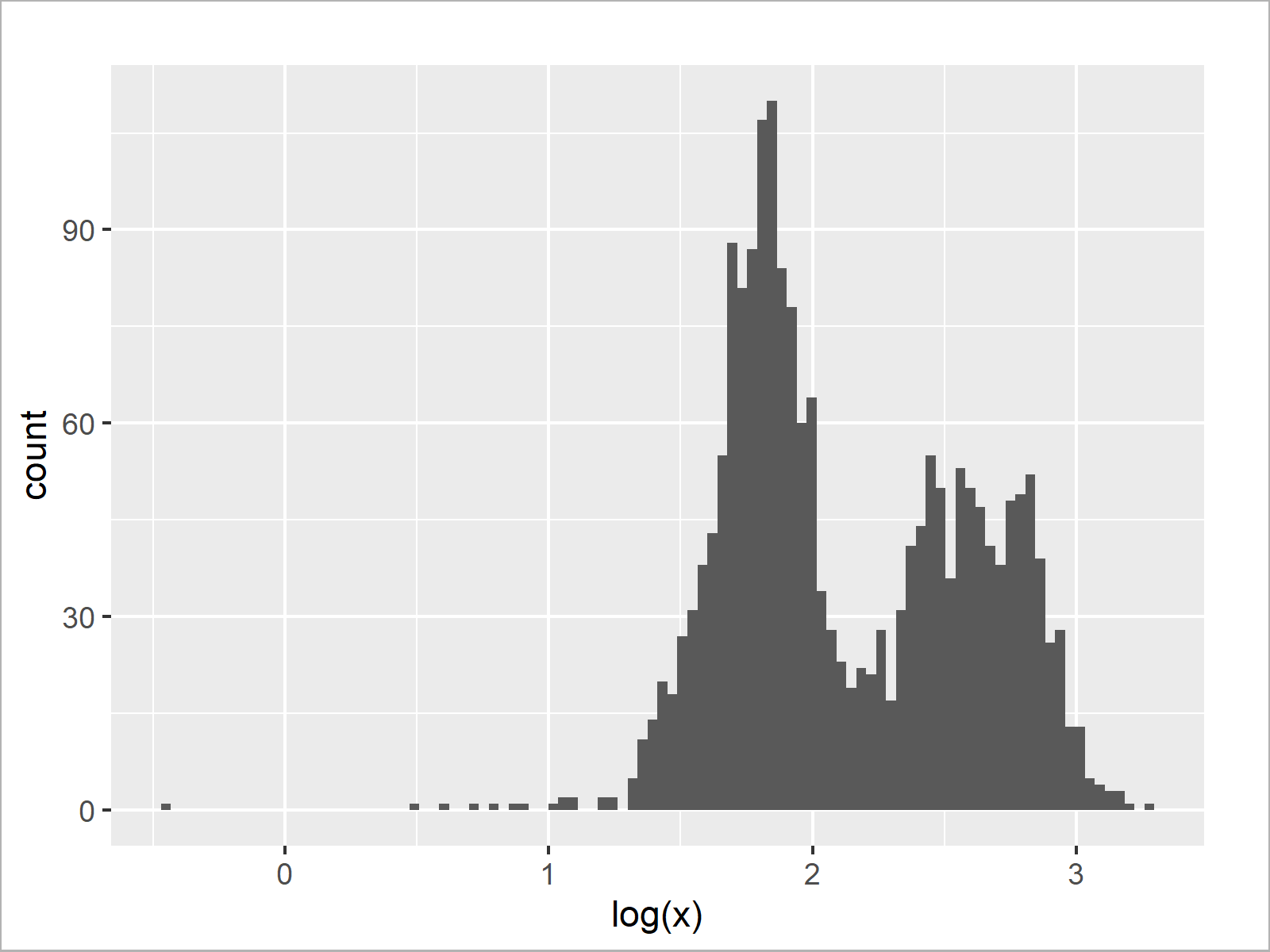# Draw Histogram with Logarithmic Scale in R (3 Examples)

This article illustrates how to convert the x-axis of a graph to log scale in R.

The article is structured as follows:

It’s time to dive into the examples:

## Creation of Example Data

Have a look at the following example data:

```set.seed(120653)                              # Set random seed
x <- c(rnorm(1000, 6), rnorm(1000, 13, 4))    # Create example data
#  6.717975 5.000511 5.836833 5.255884 5.894466 5.847968```

The previous output of the RStudio console shows the structure of our example data – It’s a numeric vector containing randomly drawn numbers.

## Example 1: Draw Histogram with Logarithmic Scale Using Base R

Example 1 shows how to create a Base R histogram with logarithmic scale.

Let’s first draw a histogram with regular values on the x-axis:

`hist(x, breaks = 100)                         # Histogram without logarithmic axis`As shown in Figure 1, the previous R programming code created a histogram without logarithmic scale.

If we want to convert the x-axis values of our histogram to logarithmic scale, we can use the log function as shown below:

`hist(log(x), breaks = 100)                    # Histogram with logarithmic axis`As shown in Figure 2, the previous syntax created a Base R histogram with logarithmic scale.

## Example 2: Draw Histogram with Logarithmic Scale Using ggplot2 Package

In this example, I’ll explain how to draw a ggplot2 histogram with logarithmic scale.

If we want to use the functions of the ggplot2 package, we first need to install and load ggplot2:

```install.packages("ggplot2")                   # Install & load ggplot2 package
library("ggplot2")```

Next, we can draw a ggplot2 histogram without log scale:

```ggplot(data.frame(x), aes(x)) +               # Histogram without logarithmic axis
geom_histogram(bins = 100)```The output of the previous R code is shown in Figure 3 – A ggplot2 histogram with default x-axis values.

Let’s transform our x-axis to logarithmic scale:

```ggplot(data.frame(log(x)), aes(log(x))) +     # Histogram with logarithmic axis
geom_histogram(bins = 100)```As shown in Figure 4, the previous code created a ggplot2 histogram with logarithmic scale using the log and geom_histogram functions.

## Example 3: Draw Histogram with Logarithmic Scale Using scale_x_log10 Function of ggplot2 Package

In case we want to draw a ggplot2 histogram with log10 scale, we can use the scale_x_log10 function instead of the log function.

```ggplot(data.frame(x), aes(x)) +               # Histogram with log10 axis
geom_histogram(bins = 100) +
scale_x_log10()```The output of the previous R programming code is shown in Figure 5 – A ggplot2 plot with log10 x-axis scale.

## Video & Further Resources

Do you need more explanations on the R programming codes of this tutorial? Then you might have a look at the following video of my YouTube channel. In the video, I’m explaining the R programming code of this article:

Please accept YouTube cookies to play this video. By accepting you will be accessing content from YouTube, a service provided by an external third party.If you accept this notice, your choice will be saved and the page will refresh.

In addition, you might want to have a look at the other articles on my website.

In this post you learned how to display the x-axis of a graphic in logarithmic scale in the R programming language. In case you have any further questions, let me know in the comments section below.

Subscribe to the Statistics Globe Newsletter

•Jack
February 7, 2023 9:48 am

Hello there, could you help me with changing x-axis values for a log scaled histogram?

I have used hist(log(mydata\$data, 10)) which gives me the histogram I want but now I want to change the x-axis values from log base exponents to the actual value they represent i.e., instead of 1, 2, 3, 4, 5 I’d like 10, 100, 1000, 10000, 100000.

•February 7, 2023 12:24 pm

Hello Jack,

I understand that you want to plot a histogram for the transformed data but use the actual values as labels on the x-axis. Then I suggest you run the code below.

```set.seed(120653)                              # Set random seed
x <- c(rnorm(1000, 6), rnorm(1000, 13, 4))    # Create example data